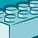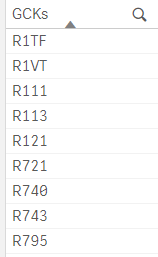# App Development

Announcements
April 22, 2PM EST: Learn about GeoOperations in Qlik Sense SaaS READ MORE
cancel
Showing results for
Did you mean:Creator

## Generate next value based on the previous value

Hello everyone,

I have the following issue if anyone can help with it, please.

i have a list of PCK numbers: as shown in table below. I want to generate something similar to the table below. My only issue is the first column. Suppose that i have the first number, how do i use the previous rows number and add 10 to it to make the next number. So, for example, my first number is 40 and for the first row i use 40+10=50. For the next iteration, i want to use 50+10=60 and so on. It is like iterative calculation using the value i pass in.

the number 40 is taken from another table. It is the max "Sort Number" in another table. so I use Peek() to obtain the max number from that table. Now i want to generate a separate table with the PCK list i have.

Please let me know if you are unsure of what i am trying to ask.

 SortNumber PN Indicator PCK GCK 50 * * R444 * 60 * * T3233 * 70 * * Y351 * 80 * * R2331 *

Thank you.

Labels (4)

• ### Qlik Sense

1 Solution

Accepted SolutionsCreator III

Another would be to have your load script this way assuming you have your starting number assigned to a variable called "vStartingNum".  I tested this in September 2019 release and it returns 6 rows with IDs 50-100 progressing by 10s.

Table1:
INLINE [
40
];

let vStartingNum = Peek('SomeNumber', 0, 'Table1');

Trace \$(vStartingNum);

NoConcatenate
Table2:
INLINE [
SomeOtherData
asdfa
sdfghdhrr
mrsrm3
mrytdmth
mydf
mhgmdgh
];

NoConcatenate
Table3:
Resident Table2;

Drop Table Table2;

10 RepliesContributor III

Set vFirstNumber = the max "Sort Number" from another table, then use this script:

``````LOAD
PCK
,if(RowNo()=1,\$(vFirstNumber),Peek('SortNumber'))+10 as SortNumber

It uses RowNo() to check if it is loading the first row. If so, it uses the vFirstNumber variable you set earlier (should be 40). After the first row, the table will load the previous SortNumber instead of using vFirstNumber.Creator
Author

Hey Gavin,

Thank you for the quick reply.  I just have one question

`if(RowNo()=1,\$(vFirstNumber),Peek('SortNumber'))+10 as SortNumber`

in the above code, you have Peek('SortNumber' ) and you are also assigning the column "as SortNumber". are you referencing the same column? or in different words, are both SortNumber colored red the same thing?

Also, i tried it and it is giving me null valuesContributor III

Yes, it is referencing the same "SortNumber" column. That is why the first part of the expression is required- "if(RowNo()=1" checks to see if it is loading the first row (in which case, there would be no "SortNumber" field yet to reference with Peek). For the first row, it uses \$(vFirstNumber), rather than Peek('SortNumber'). For all rows after that, it uses Peek('SortNumber') since the "SortNumber" field exists at that point.Creator
AuthorI am getting the following result using your codeContributor IIICreator III

Another would be to have your load script this way assuming you have your starting number assigned to a variable called "vStartingNum".  I tested this in September 2019 release and it returns 6 rows with IDs 50-100 progressing by 10s.

Table1:
INLINE [
40
];

let vStartingNum = Peek('SomeNumber', 0, 'Table1');

Trace \$(vStartingNum);

NoConcatenate
Table2:
INLINE [
SomeOtherData
asdfa
sdfghdhrr
mrsrm3
mrytdmth
mydf
mhgmdgh
];

NoConcatenate
Table3:
Resident Table2;

Drop Table Table2;Creator
Author
``````let vSortNumber = Peek('Sort Number',-1,'Derivation_Rule_Table'); //8640

Temp:

if(RowNo()=1,\$(vSortNumber),Peek('SortNumber'))+10 as SortNumber,
;
Load Distinct(FGCK) as GCKs //there are only 9 distinct GCK values
Resident Material_Master_Table

The result of this is as follows:it took away the distinct GCKs and duplicated it over and over again(thousands of records). I am expecting only 9 rows starting from 8650 til 8740 as sort number since there are only 9 distinct GCKs. the above snippet actually starts from 8650 til 25480 (shown above)MVP

would you be able to share sample data?Creator
Author

Hello Kush,

for sure. I have the following GCK values:and what is want is the following:For each of the GCK values, i want ONE ROW only. with the values similar to above ( which i can hard code into the script). the only issue is getting a number that is the incremented by 10 from the previous value. So, in the case above, first value is 8650, 8660,8670,8680 and so on.